Latest Banking jobs   »   Quantitative Aptitude Quiz For IDBI AM/Executive...

# Quantitative Aptitude Quiz For IDBI AM/Executive 2022- 19th June

Directions (1-5): Given below is the pie chart which shows the distribution of six books sold by bankersadda publication in degrees in one week. Table shows ratio of books sold online to sold from book store.

(Note – Sold DI books in one week in absolute value)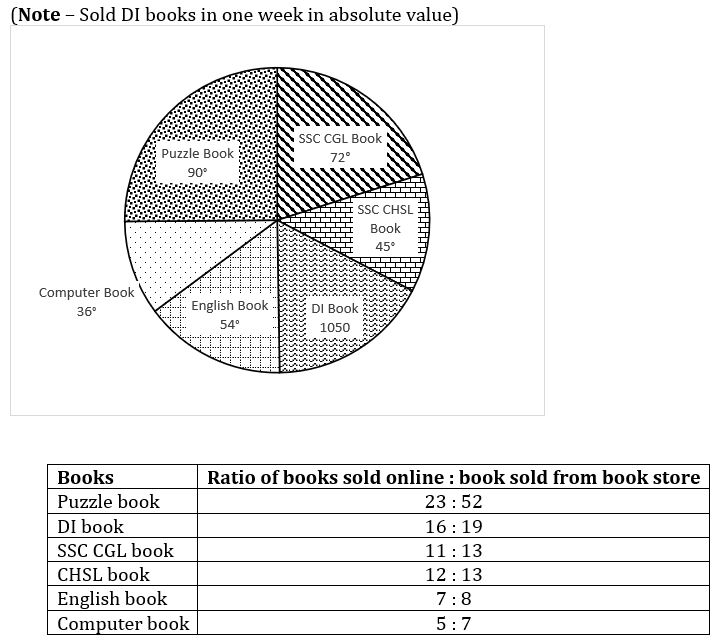Q1. If selling price of each SSC CHSL books is 11(1/9)% less than the selling price of each English book, and total amount obtained in selling all SSC CHSL book is 1.5 lakh. Then find how much amount is obtained in selling all English books, which sold online ?
(a) 96000 Rs.
(b) 94000 Rs.
(c) 94500 Rs.
(d) 95400 Rs.
(e) 94540 Rs.

Q2. Total DI book and Puzzle book sold online is what percent less or more than total computer and English books sold from store ?
(a) 11 (21/83)%
(b) 12 (21/83)%
(c) 15 (21/83)%
(d) 14 (21/83)%
(e) 13 (21/83)%
Q3. If 25% of Puzzle books and 20% of DI books sold from book store is returned by customers, because of misprinting, then find the number of DI and PUZZLE book which are returned by customers ?
(a) 347
(b) 374
(c) 376
(d) 367
(e) 370

Q4. What is ratio between, Puzzle and DI books sold together to SSC CHSL, SSC CGL and English book sold together ?
(a)17:15
(b)17:21
(c)17:18
(d)17:19
(e)19:17

Q5. Total Computer and DI book sold online together, what percent of total SSC CGL book sold from store ?
(a)112 (4/13)%
(b)113 (4/13)%
(c)110 (4/13)%
(d)111 (4/13)%
(e)109 (4/13)%

Directions (6-10): The following questions are accompanied by three statements (I), (II), and (III). You have to determine which statements(s) is/are sufficient/necessary to answer the questions.
Q6. Making a new t-shirt, consists of two parts first is cutting of cloth and second is stitching the cloth. In how many days Nitin and Vishal together can make 100 t-shirts.
(I) Nitin can cut cloth for 20 t-shirts in a day and he is 20% slow as that of Vishal in cutting clothes and 25% faster than Vishal for stitching the cloth.
(II) time taken by Nitin alone to make 60 t-shirts is 9 days.
(III) The ratio of days taken by Vishal to cut cloth for 50 t-shirts and to stich 100 t-shirts is 4 : 25.
(a) Only I and III together or only II and III together
(b) All I, II and III together
(c) Any two of the three
(d) Only I and III together
(e) Either from I & II or I & III

Q7. Ratio of age of Aashish & Kullu is 4 : 5 and that of Nikhil and Yash is 7 : 8. What is their average age after 4 years.
(I) Age difference of Nikhil and Aashish is 18 years.
(II) Ratio of 12 years ago age of Aashish & Nikhil is 2 : 5 and Kullu & Yash is 1 : 2.
(III) 12 years later Aashish will be 150% of his present age.
(a) Any two of I, II and III are required.
(b) Only I and II are required
(c) Only II and III are required
(d) All I, II and III together are required
(e) II alone or from I and III are required

Q8. Three athletes practice running on a circular track of 400 m. who runs fastest among them?
(I) when A starts running clockwise and B starts running anticlockwise, they meet 2nd time at a distance of 57 (1/7) meter from starting point in clockwise direction after 22 (6/7) seconds.
(II) B runs 5 m/s faster than C. A is not twice or more fast than B and neither B is twice or more fast than A or C.
(III) when A, C and B, all runs in same direction, A and C only meet at starting point, while ratio of speed of B to C is 3 : 2.
(a) Any one of them
(b) Only I and II together are sufficient
(c) Any two of the three together are sufficient
(d) None of the above
(e)all the three statements are not sufficient.

Q9. A man has 16 balls with him, in 3 colors i.e. Red, green and blue. Three balls are drawn at random, what is the probability that all are blue.
(I) the probability of drawing a blue ball is greater than 1/8 and less than 5/16. Also, the number of green balls he has is odd and number of red balls he has is even.
(II) Probability of drawing red ball is 3/8 which is 1/16 lesser than that of green balls.
(III) If a man lost one ball, probability of drawing a blue ball is ⅓.
(a) Only I and III or only II and III
(b) All I, II and III together
(c) Any one of them is sufficient
(d) Only I and III together sufficient
(e) None of the above

Q10. A man bought two bats and 6 identical balls, he sold all of them in a day, calculate his overall profit %.
(I) He sold one bat at a price of Rs. 600 and other at Rs. 420 and profit is 20% on both bats. Each ball is sold at 12% profit.
(II) Profit earned from 6 balls is Rs. 36 and profit earned from both bats is Rs. 170.
(III) Profit earned on each bat is 20% while cost price of each ball is Rs. 50.
(a) Any one of them
(b) Only I and II together are sufficient
(c) Any two of the three together are sufficient
(d) None of the above
(e) All three together are sufficient

Q11. Arun and Rahul started a business together. Rahul invested Rs.20,000 more than Arun and left 4 months before completion of year. Calculate total money invested by Rahul (in Rs.), if after a year Arun’s profit share is Rs. 22500 which is 1500 more than that of Rahul.
(a) 50000
(b) 60000
(c) 40000
(d) 70000
(e) 55000

Q12. Ratio of present age of A and B is 4 : 5, while that of C and D is 6 : 7. If 10 years ago Ratio of age of A and C is 1 : 2 and that of B & D is 3 : 5, Find average of present age of A, B and C together.
(a) 27.5
(b) 25
(c) 27
(d) 26.5
(e) 24.5

Q13. A shopkeeper has leather and tennis balls. If he sold 10 leather balls at 10% profit and 8 tennis balls at 12.5% profit, then total profit earned by him is Rs. 720. If selling price of tennis ball is Rs. 98 more than that of leather ball then calculate the S.P. at which shopkeeper was selling each leather balls.
(a) Rs.325
(b) Can’t be determined
(c) Rs.400
(d) Rs.352
(e) None of these

Q14. Two trains A and B of length x and y meters, are travelling with speed y km/hr and x km/hr. Calculate time taken by them to cross each other, while travelling in opposite direction.
(a) 0.24 second
(b) 0.27 second
(c) 3.6 seconds
(d) Can’t be determined
(e) None of these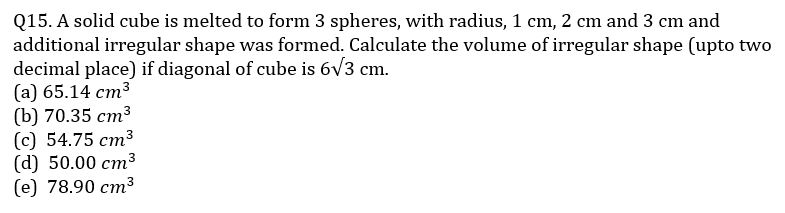Solutions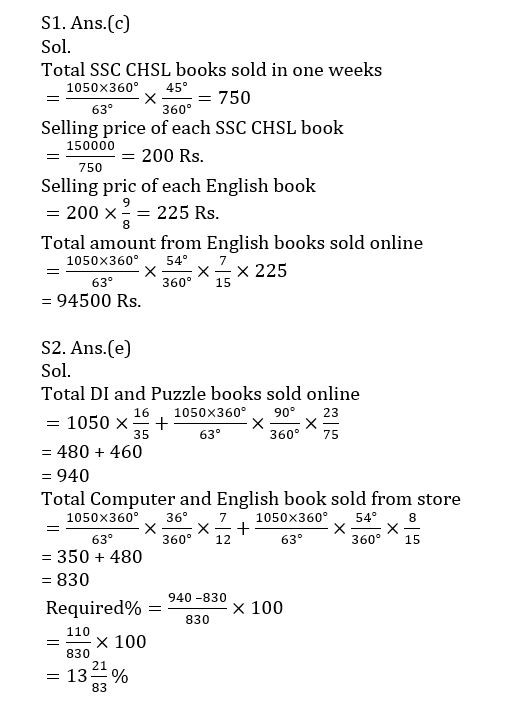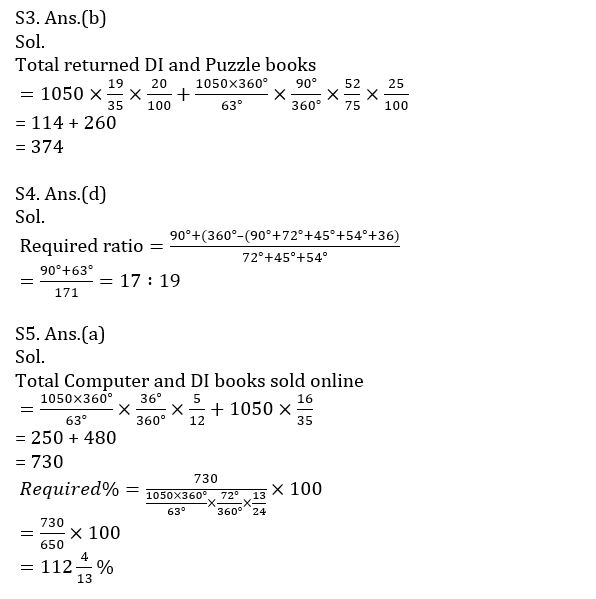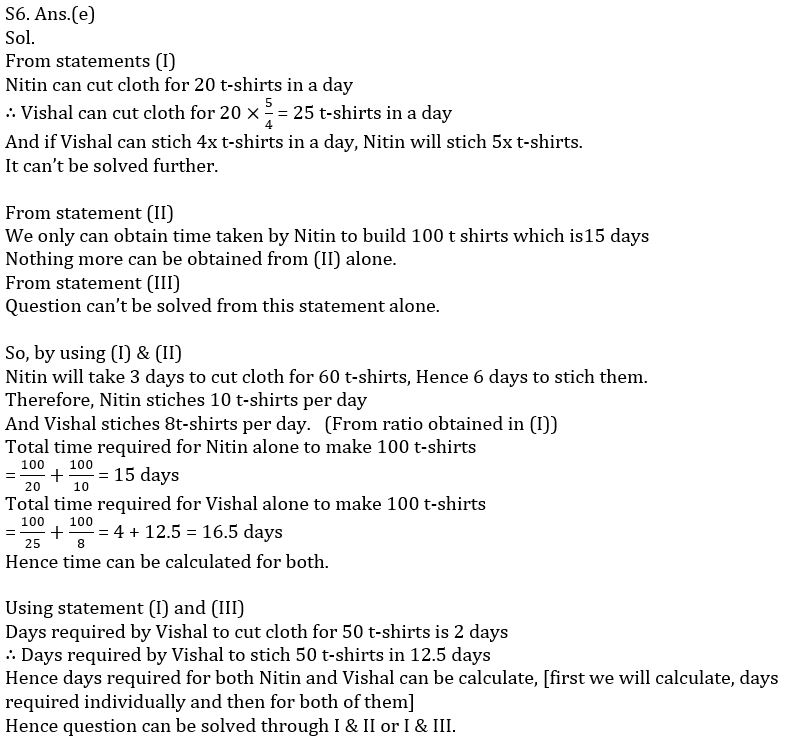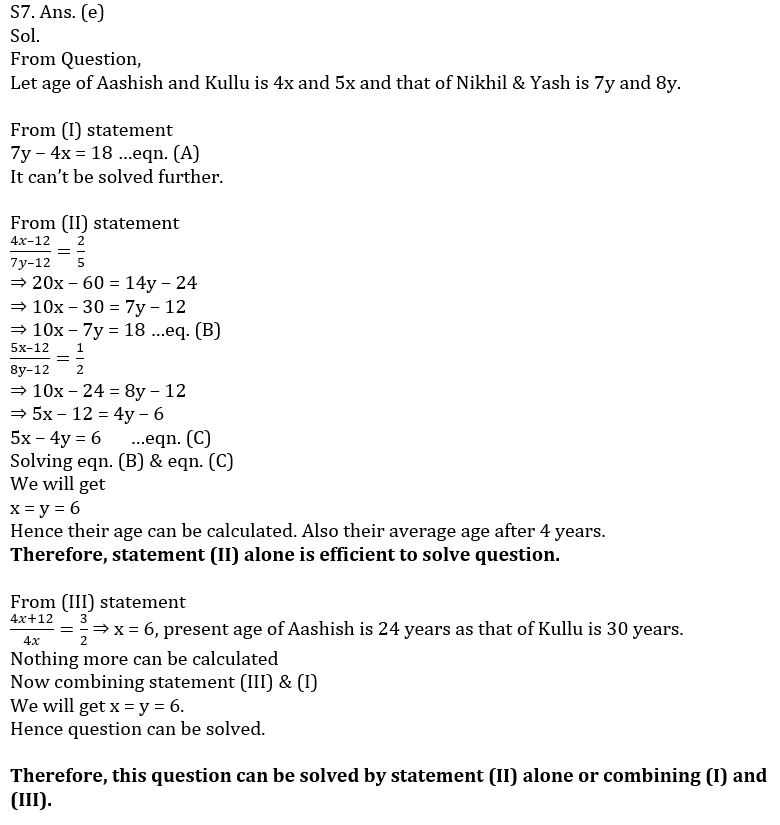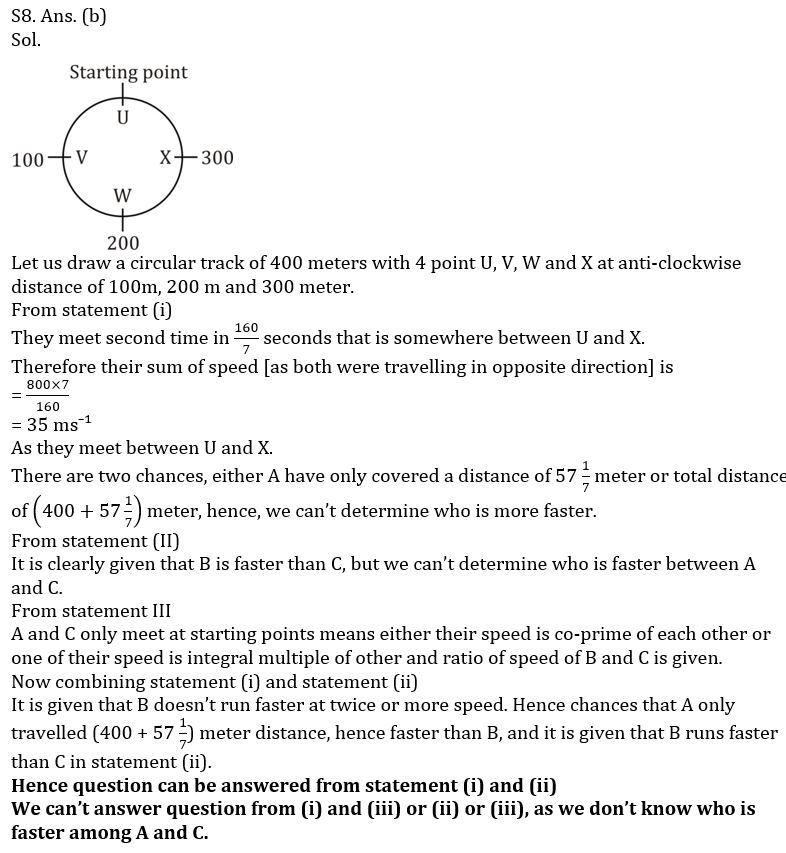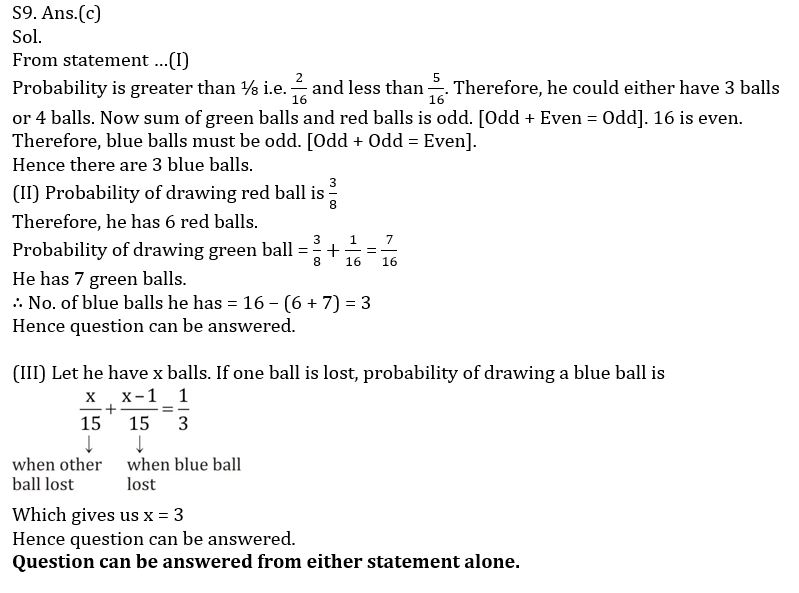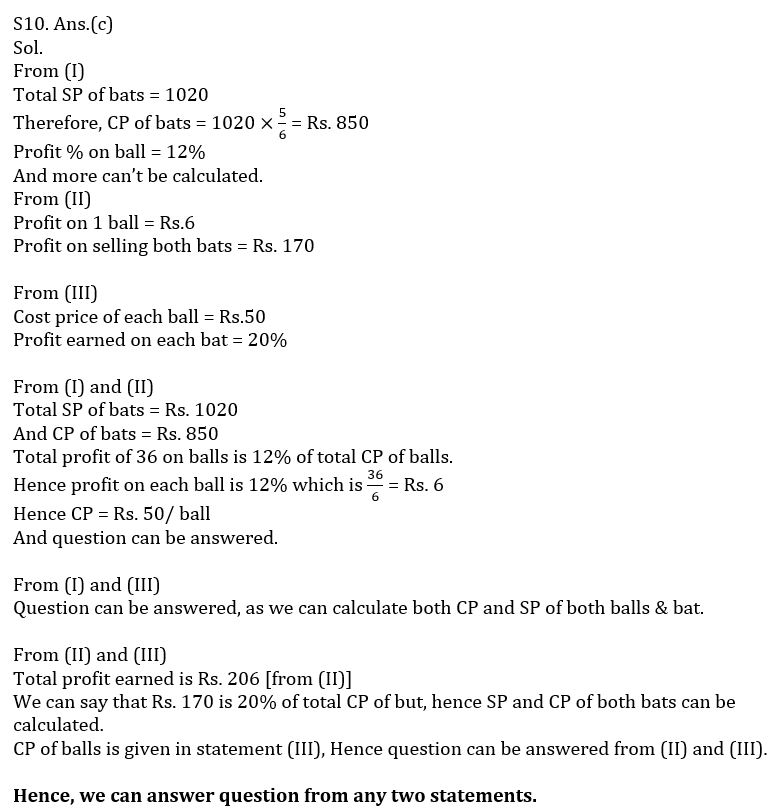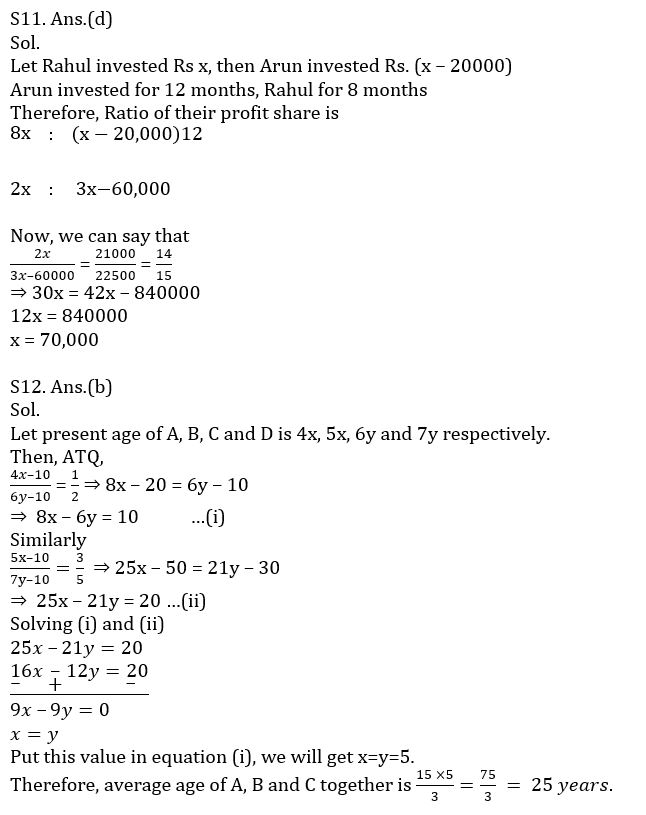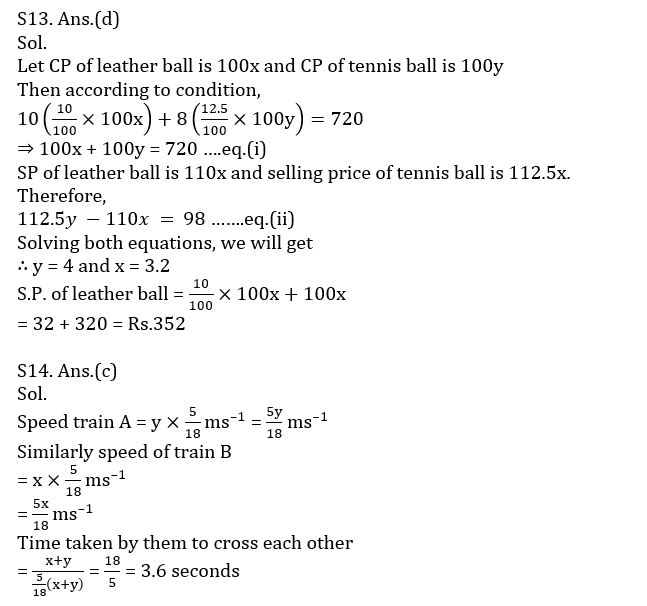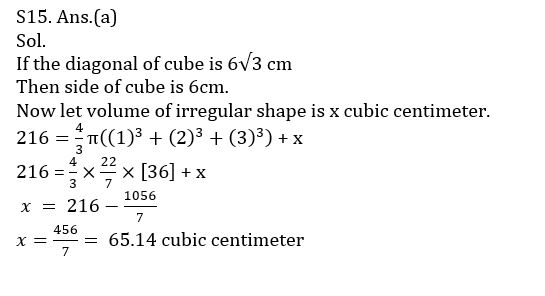#### Congratulations!